# Imaging and Design for the Online Environment - Empowerment Technologies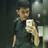Former College and SHS Instructor at ACES Polytechnic College em ACES Polytechnic College
14 de Dec de 2017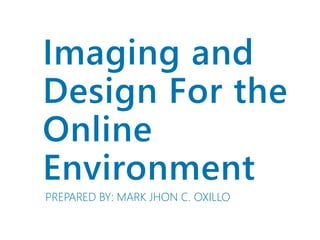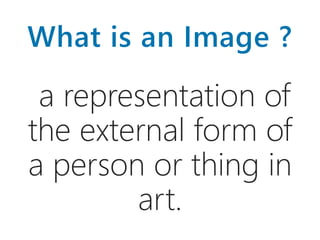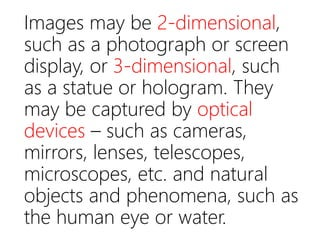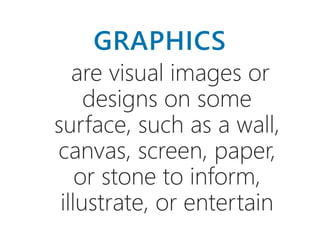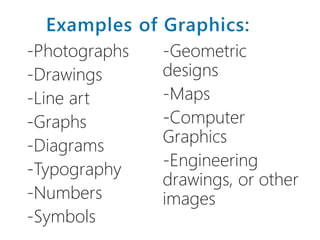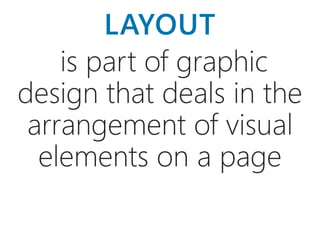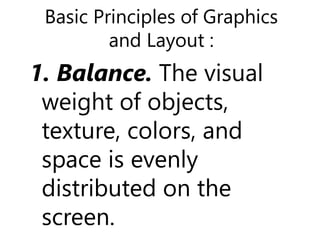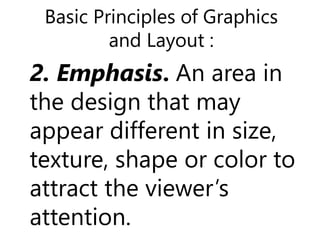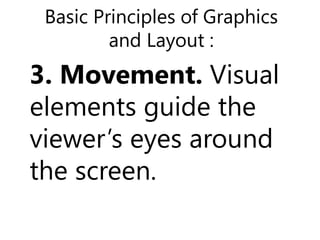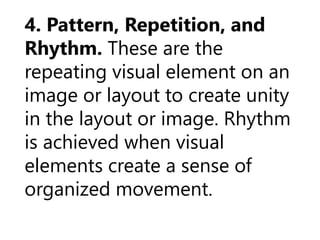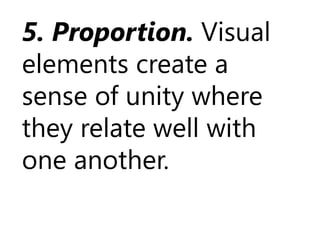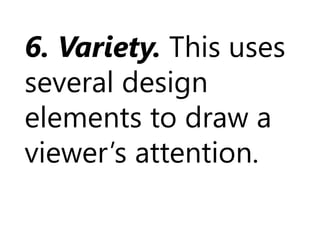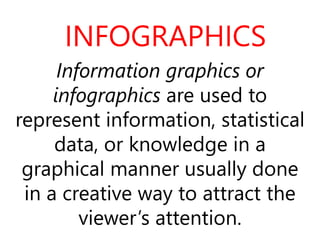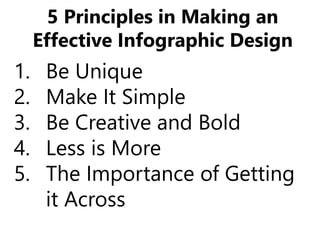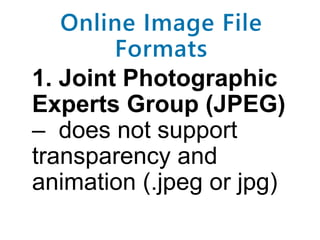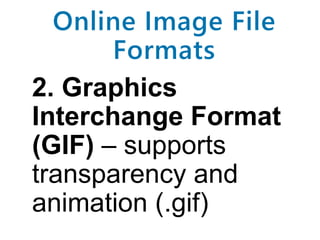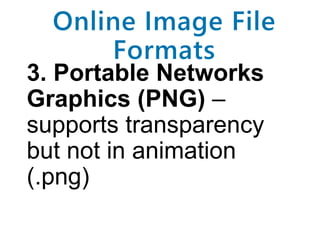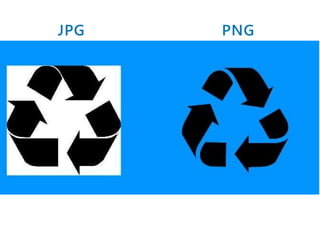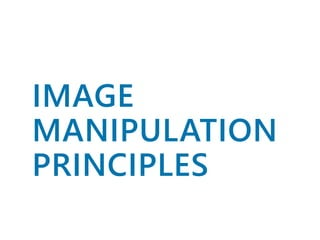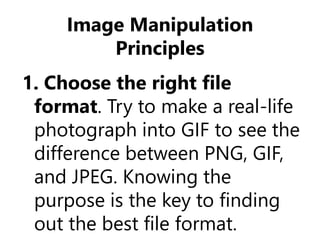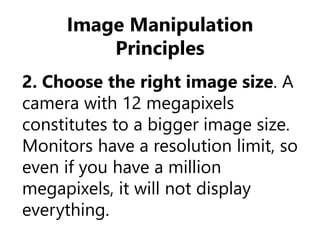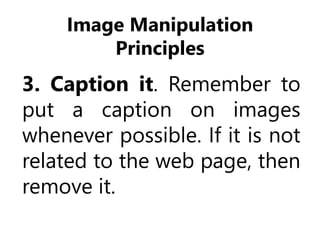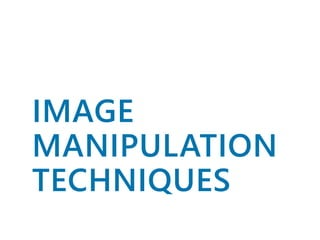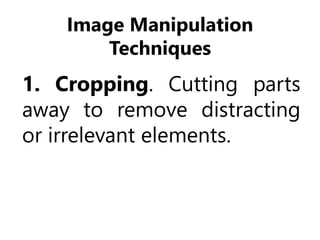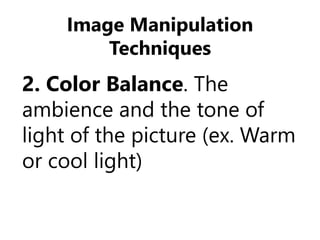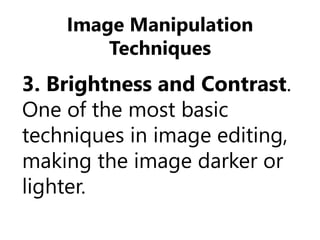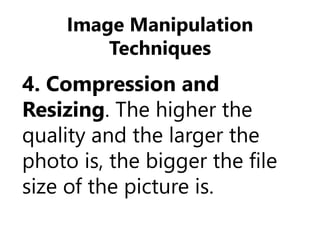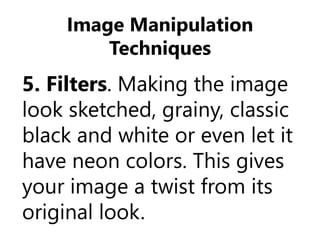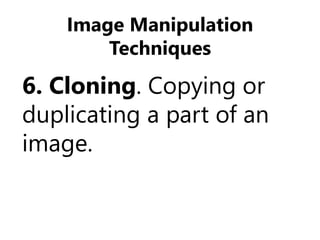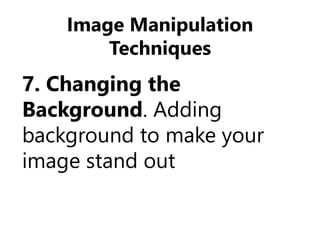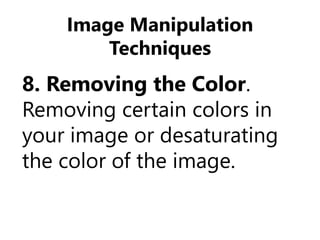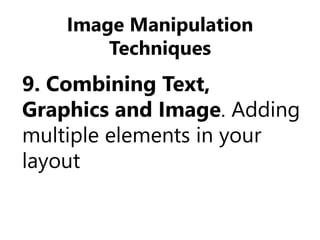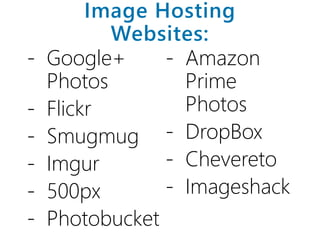1 de 35

### Notas do Editor

1. Practicum. Ask the students to manipulate an image using the tips provided in the slide.
2. Practicum. Ask the students to manipulate an image using the tips provided in the slide.
3. Practicum. Ask the students to manipulate an image using the tips provided in the slide.
4. Practicum. Ask the students to manipulate an image using the tips provided in the slide.
5. Practicum. Ask the students to manipulate an image using the tips provided in the slide.
6. Practicum. Ask the students to manipulate an image using the tips provided in the slide.
7. Practicum. Ask the students to manipulate an image using the tips provided in the slide.
8. Practicum. Ask the students to manipulate an image using the tips provided in the slide.
9. Practicum. Ask the students to manipulate an image using the tips provided in the slide.
10. Practicum. Ask the students to manipulate an image using the tips provided in the slide.
11. Practicum. Ask the students to manipulate an image using the tips provided in the slide.
12. Practicum. Ask the students to manipulate an image using the tips provided in the slide.